### Geometry Fundamentals

Is there more area shaded pink or blue?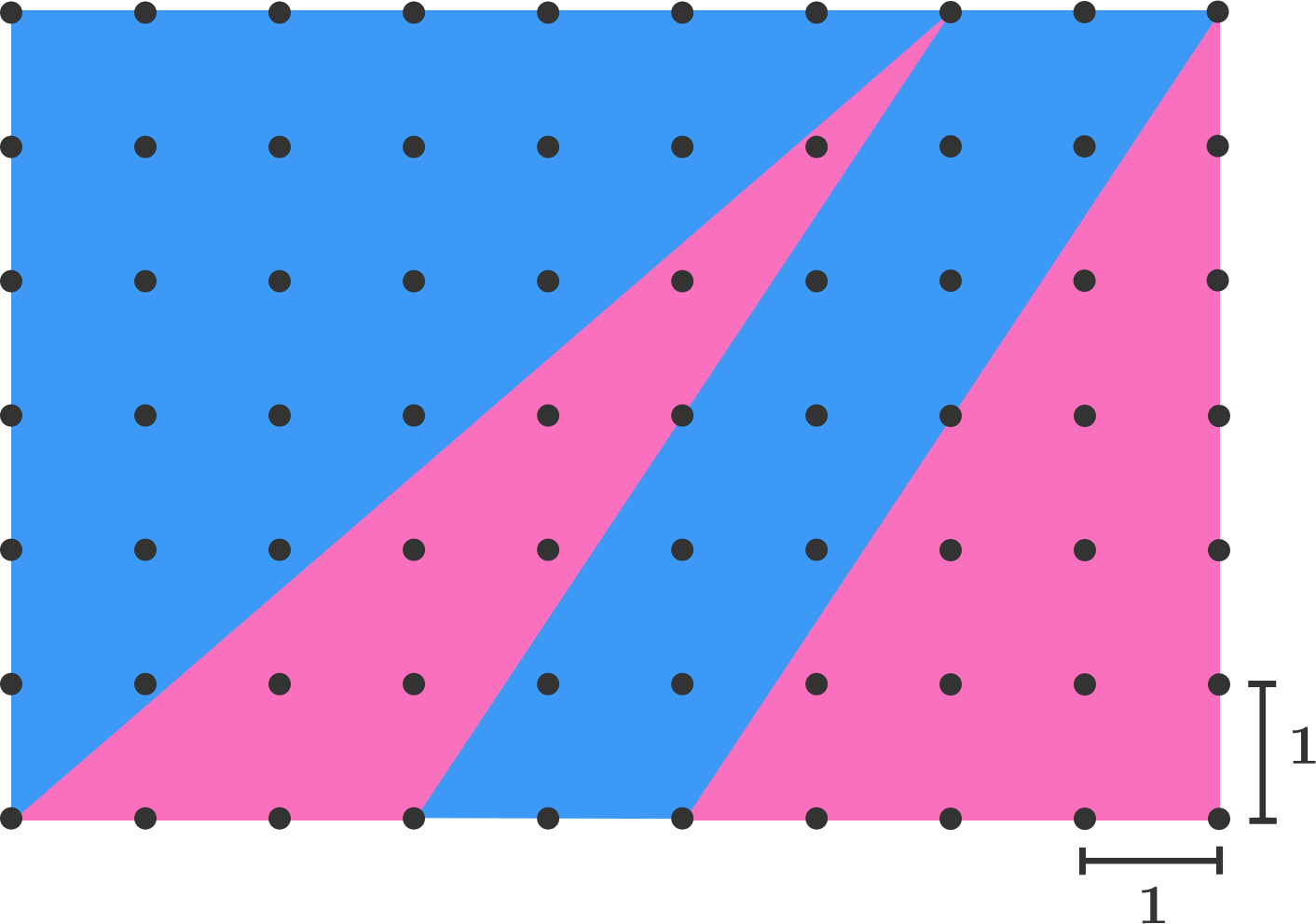# Exploring Area

Strategy can be more important than formulas when solving problems. By cutting and/or rearranging pieces, we can compare areas intuitively and efficiently.

As you move through the next several problems, continue to use creative strategies and your intuition about shapes to find solutions.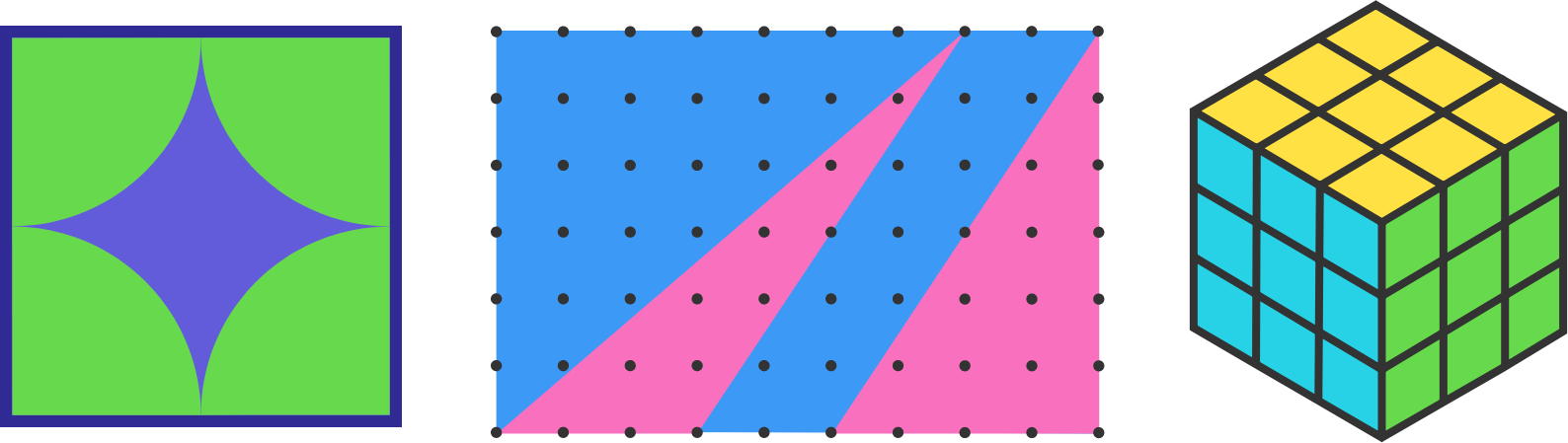# Exploring Area

Given that figures A, B, and C have the same dimensions, in which figure is the area of the regions shaded purple the greatest?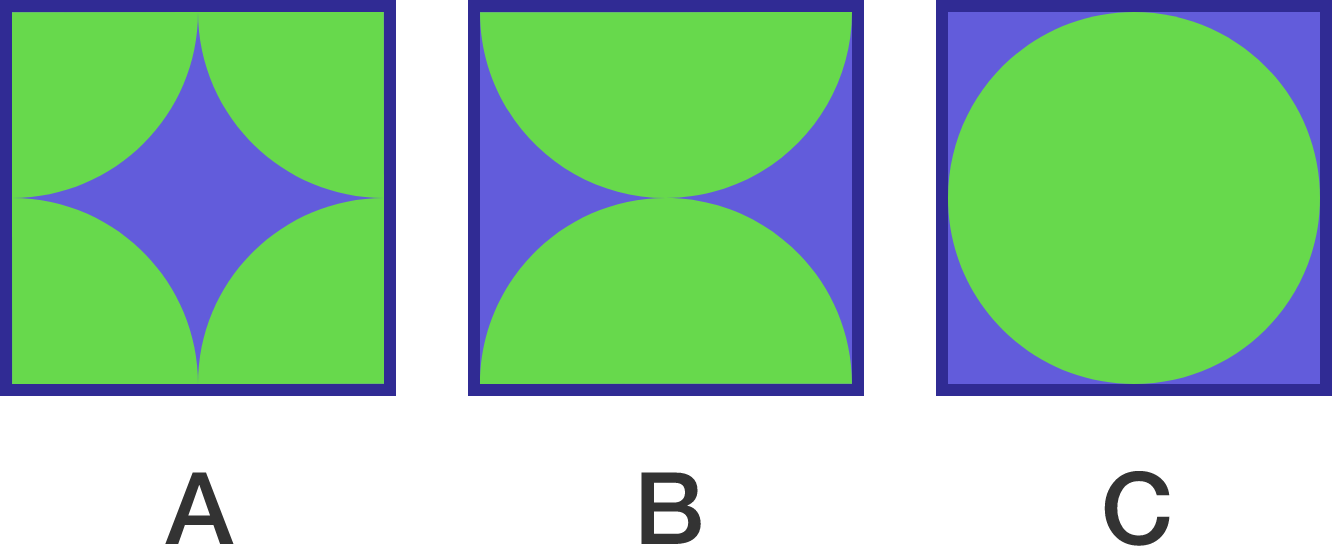Note: The green quarter-circles of A, green half-circles of B, and green full circle of C all have the same radius.

# Exploring Area

The figure consists of 6 unit squares. What is the area of the portion shaded green?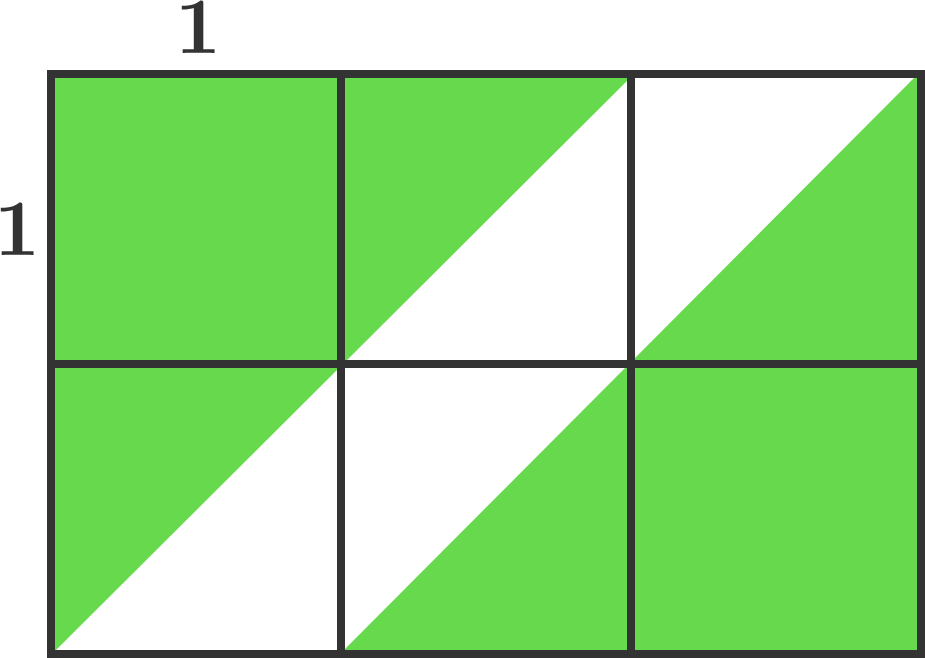# Exploring Area

The large triangles are identical, and the small triangles within a large triangle are also identical. Which is larger, the green area on the left or the purple area on the right?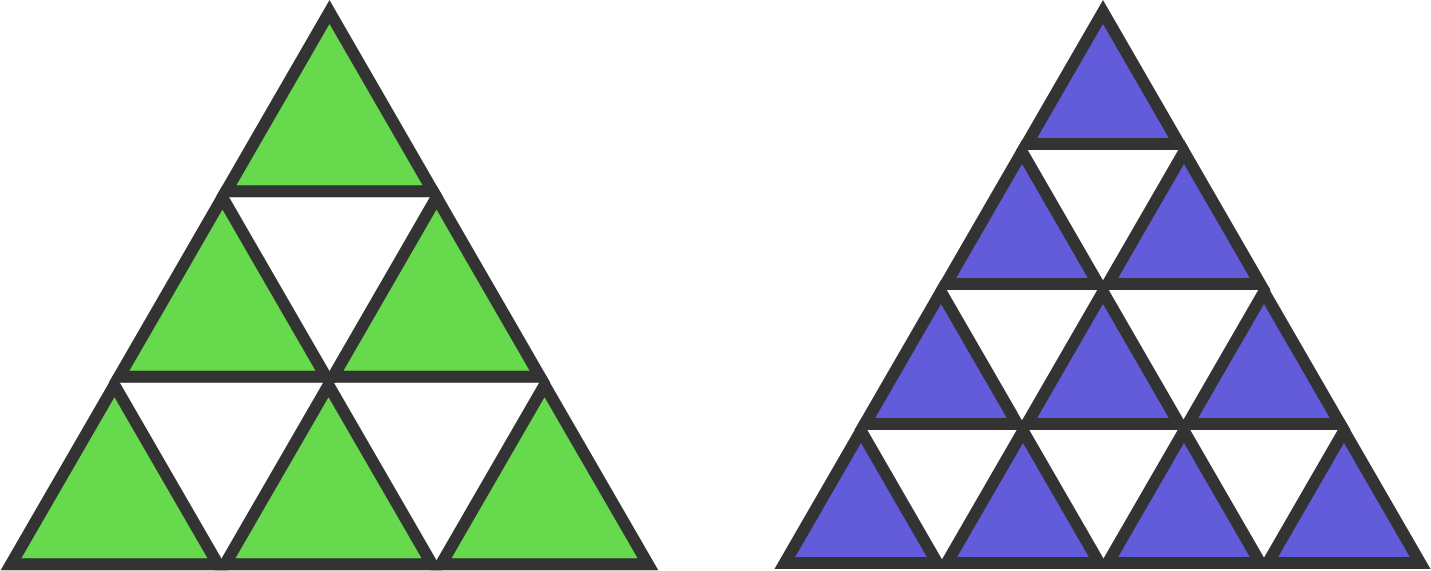# Exploring Area

The area of the large square is 100 and all of the small, blue squares are congruent.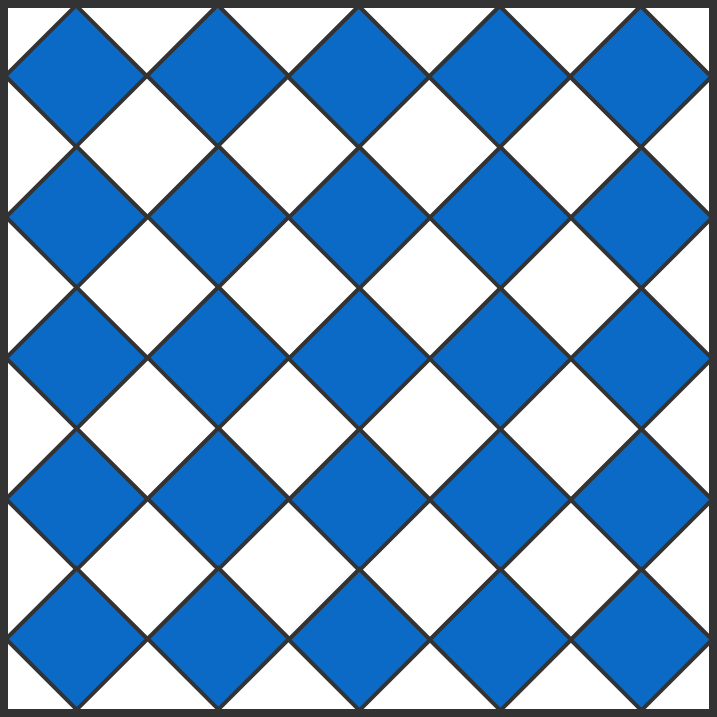What is the total area shaded blue?

×